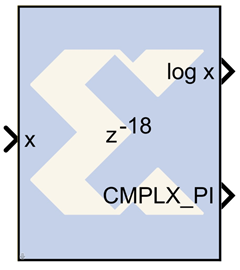# CORDIC LOG - 2020.2 English

## Vivado Design Suite Reference Guide: Model-Based DSP Design Using System Generator (UG958)

Document ID
UG958
Release Date
2020-11-18
Version
2020.2 English

The Xilinx CORDIC LOG reference block implements a natural logarithm circuit using a fully parallel CORDIC (COordinate Rotation DIgital Computer) algorithm in Hyperbolic Vectoring mode.That is, given a input x, it computes the output log (x) and also provides a flag for adding complex pi value to the output if a complex output is desired. The CORDIC processor is implemented using building blocks from the Xilinx blockset.

The natural logarithm is calculated indirectly by the CORDIC algorithm by applying the identities listed below.

log (w) = 2 x tanh-1[(w-1) / (w+1)]

log ( w x 2E ) = log (w) + E x log (2)

The CORDIC LOG algorithm is implemented in the following 4 steps:

1. Co-ordinate Rotation: The CORDIC algorithm converges only for positive values of x. If x < zero, the input data is converted to a non-negative number. If x = 0, a zero detect flag is passed along to the last stage which can be exposed at the output stage. The log circuit has been designed to converge for all values of x, except for the most negative value.
2. Normalization: The CORDIC algorithm converges only for x, between the values 0.5 (inclusive) and 1. During normalization, the input X is shifted to the left till it has a 1 in the most significant bit. The log output is derived using the identity log(w) = 2 x tanh-1{ (w-l) / (w+1) }. Based on this identity, the input w gets mapped to, x = w + 1 and y = w - 1.
3. Linear Rotations: For tanh-1{(w-l) / (w+1)} calculation, the resulting vector is rotated through progressively smaller angles, such that y goes to zero.
4. Co-ordinate Correction: If the input was negative a CMPLX_PI flag is provided at the output for adding PI if a complex output is desired. If a left shift was applied to X, this step adjusts the output by using the equation log (w x 2E) = log (w) + E x log (2).

## Block Parameters

The block parameters dialog box can be invoked by double-clicking the icon in your Simulink model.

Parameters specific to this reference block are as follows:

• Number of Processing Elements (integer value starting from 1): specifies the number of iterative stages used for hyperbolic rotation.
• Input Data Width: specifies the width of input x. The inputs x should be signed data type having the same data width.
• Input Binary Point Position: specifies the binary point position for input x. The input x should be signed data type with the same binary point position.
• Latency for each Processing Element : This parameter sets the pipeline latency after each circular rotation stage.

The latency of the CORDIC LOG block is calculated based on the formula specified as follows: Latency = 2+ Data Width+sum (latency of Processing Elements).

## Reference

1. J. E. Volder, The CORDIC Trigonometric Computing Technique, IRE Trans. On Electronic Computers, Vol. EC-8, 1959, pp. 330-334.
2. J. S. Walther, A Unified Algorithm for Elementary Functions, Spring Joint Computer Conference (1971) pp. 379-385.
3. Yu Hen Hu, CORDIC-Based VLSI Architectures for Digital Signal Processing, IEEE Signal Processing Magazine, pp. 17-34, July 1992.